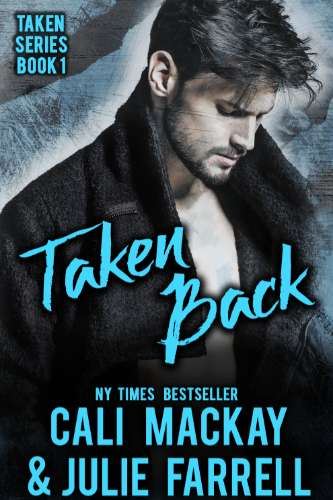A Steamy M/M Romance

Author Name: Cali MacKay & Julie Farrell
Book Name: Taken Back
Series: (Taken Series, #1)
Release Date: July 27, 2016
Publisher: Tipsy Goat Press
Cover Artist: Cali MacKay
Pages or Words: 48000 words
Categories: M/M Romance, Fiction

=•=•=•=•=•=•=•=•=•=•=•=•=•=•=•=•=•=•=•=•=•=•=•=•=•=•=•=•=Blurb

Sometimes you need to go back to your past, in order to move on to your future.

Jax Hunter has spent the last ten years trying to forget his first love Nathan Steele—and the brutal betrayal that derailed everything he’d worked so hard for. Jax has finally gotten his life back together, when Nathan returns to deliver another blow – his company is set to bulldoze the bar he’s built from nothing.

Ever since that summer in high school, Nathan has been denying his feelings for Jax—the only person he’s ever loved. Nathan knows Jax might never forgive him, but when Nathan’s uncle is murdered and Nathan might be next on the hit-list, Jax is the only one he can turn to.

Jax still doesn’t trust Nathan—not with his bar, and for damn sure, not with his heart. But the more time they spend together, the harder it is to resist the undeniable passion between them. Can they overcome their past hurts and learn to trust each other again? Or will another betrayal tear them apart forever?
=•=•=•=•=•=•=•=•=•=•=•=•=•=•=•=•=•=•=•=•=•=•=•=•=•=•=•=•=

=•=•=•=•=•=•=•=•=•=•=•=•=•=•=•=•=•=•=•=•=•=•=•=•=•=•=•=•=

An E-Copy of Taken Back

=•=•=•=•=•=•=•=•=•=•=•=•=•=•=•=•=•=•=•=•=•=•=•=•=•=•=•=•=

Meet Cali MacKay & Julie Farrell

Cali MacKay and Julie Farrell are romance writers and long-time friends, who have combined their passion for M/M romance to write together, bringing you the steamy stories they've dreamed up. they've had a blast writing them, and hope their passion for the stories will come through in their writing, and you'll enjoy the characters they've brought to life, just as much as they do.

Where to find the authors:

1 comment:

1.Thanks so much for being part of Taken Back Release Blitz